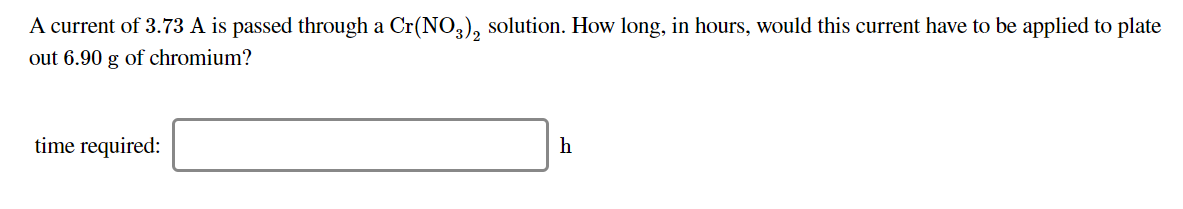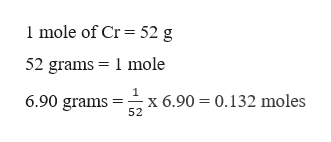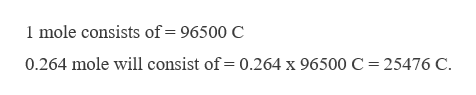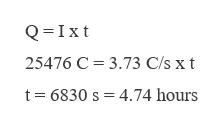# A current of 3.73 A is passed through a Cr(NO,), solution. How long, in hours, would this current have to be applied to plateout 6.90 g of chromium?time required:

Question
1 viewshelp_outlineImage TranscriptioncloseA current of 3.73 A is passed through a Cr(NO,), solution. How long, in hours, would this current have to be applied to plate out 6.90 g of chromium? time required: fullscreen
check_circle

Step 1

The charge on Cr is +2. Hence 2 moles of electrons are required,help_outlineImage Transcriptionclose1 mole of Cr = 52 g 52 grams = 1 mole =-x 6.90 = 0.132 moles 52 6.90 grams fullscreen
Step 2

1 mole of Cr requires 2 mole electrons; therefore 0.132 mole will require,

Moles of electron = 2 x 0.132 = 0.264 moles.

Now calculating the total charge present in 0.264 moles of electrons, this can be done as,help_outlineImage Transcriptionclose1 mole consists of=96500 C 0.264 mole will consist of= 0.264 x 96500 C = 25476 C. fullscreen
Step 3

The time can be cal...help_outlineImage TranscriptioncloseQ=Ixt 25476 C = 3.73 C/s x t t= 6830 s = 4.74 hours fullscreen

### Want to see the full answer?

See Solution

#### Want to see this answer and more?

Solutions are written by subject experts who are available 24/7. Questions are typically answered within 1 hour.*

See Solution
*Response times may vary by subject and question.
Tagged in

### Electrochemistry